# 国标_硅酸铝、硅酸铝板、硅酸铝毯，性价比高

## 诚信档案

### 企业名片

【友情提示】：来电请说明在中国保温防水网看到我们的，谢谢！

≥100 平方米

100000 平方米

## r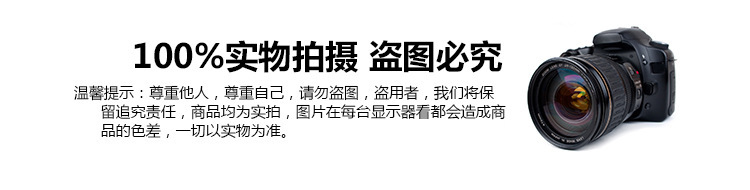r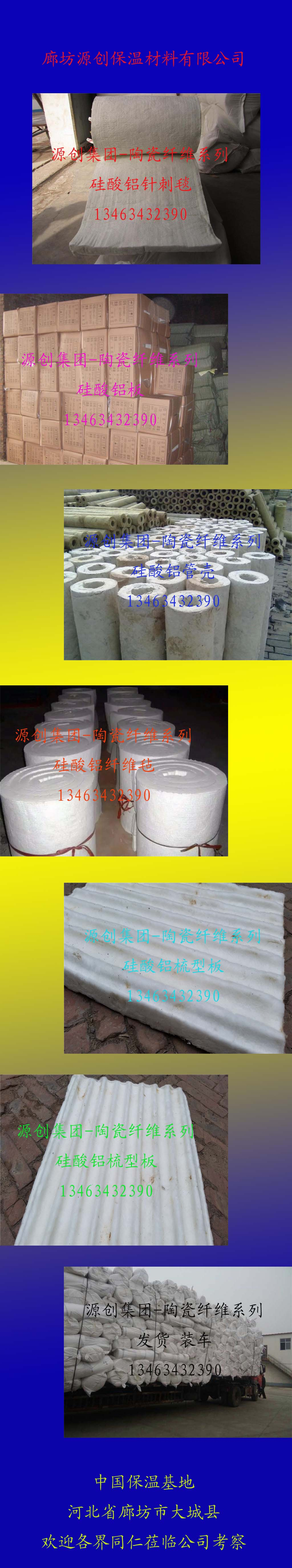r r 祁源硅酸铝制品r

r

r 硅酸铝板由精选优质焦宝石经2000℃以上电炉熔化，通过机械喷吹成纤维并均匀加入特制的粘结剂，防油剂，憎水剂经加热固化而成。主要用于电力工业、电力锅炉、汽轮机及核电隔热、船舶工业的防火隔热、建筑业、防火门的防火隔热、化工工业高温反应设备及加热设备的壁衬、汽车、火车制造业、防火、隔热、窑炉砌衬、炉门、顶盖密。
r   硅酸铝板的体系结构主要由：粘结层、保温层、抹面层、饰面层及配件。
r   粘接层为建筑归于，是介于底层和面层之间，首要用胶凝材料将上下两层牢牢粘接在一起，其填充物主要来源与无机物。
r   保温层为避免和削减汽轮机的热量向环境流失，在汽轮机及管道等外外表敷设的保温材料层，其主要填充物为焦宝石纤维以及一定量的有机物，水分，还有粘合剂等。
r   饰面层应选用饰面砂浆、点缀灰浆等轻质功能性涂层或有杰出透气性的水性外墙涂料，这样让硅酸铝板保持了其轻质的特性，也增加了其美观度。
r   配件主要选用各类涂料，一方面增加硅酸铝板的表面的颜色，让其可以适用于任何环境还有涂料可以一定程度上其到阻燃以及保温的作用。
r

r

## r  r

r

r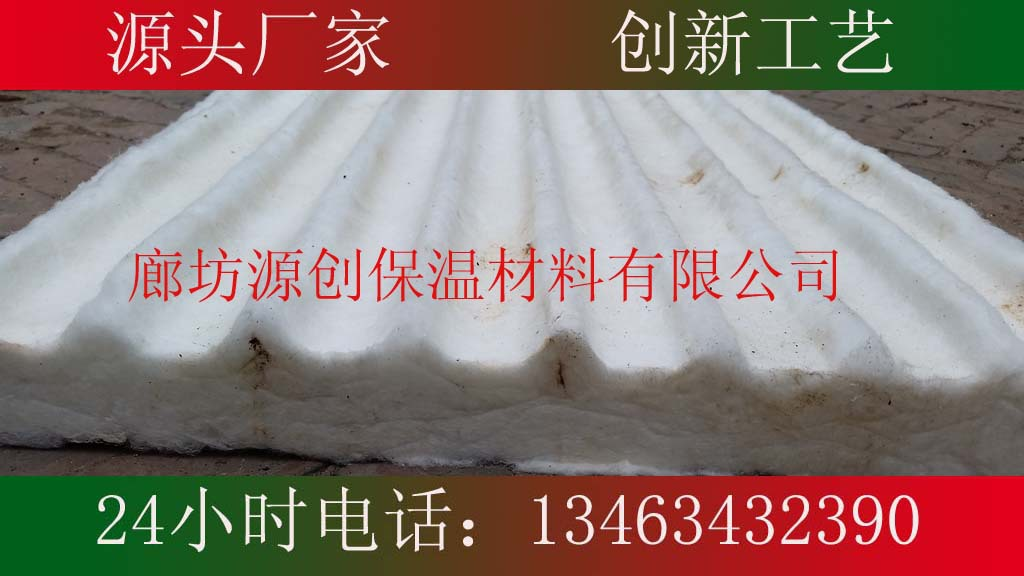r
r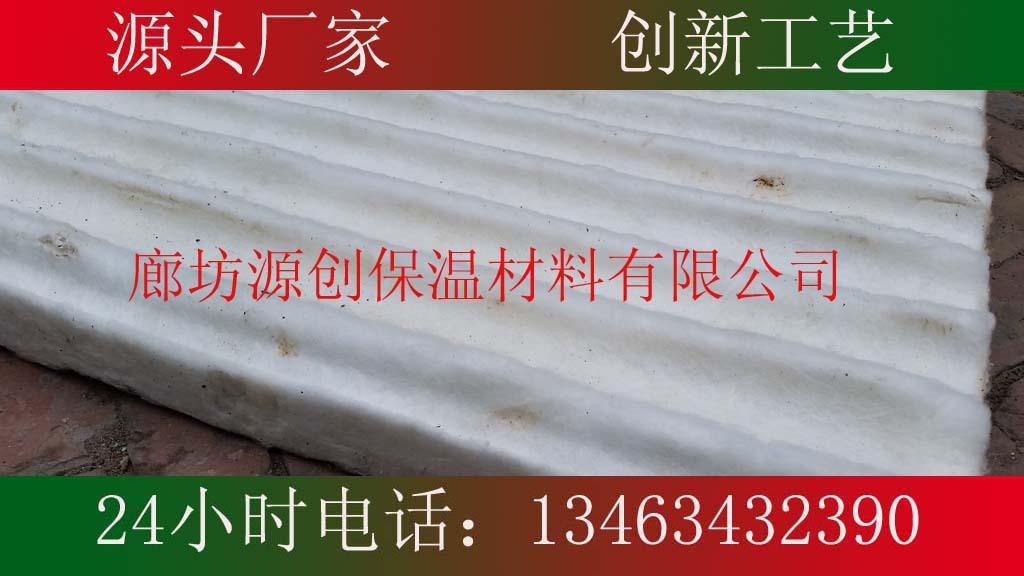r
r
r

r r r r r r r r r r r r r r r r r r r r r r r r r r r r r r r r r r r r r r r r r r r r
 r r 项目r r r r 普通硅酸铝板r r r r 高密度硅酸铝板r r r r 颜色r r r r 白r r r r 白r r r r 体积密度（kg/m）r r r r 150（±5）r r r r 170-600（±5）r r r r 备热面下导热系数r r r （w/m.k）r r r r 0.035(20℃)r r r 0.09(400℃)r r r 0.126(600℃)r r r 0.159(800℃)r r r r 0.035(20℃)r r r 0.085(400℃)r r r 0.124(600℃)r r r 0.16(800℃)r r r r 永久线收缩（%）r r r （保温24小时）r r r r -4.0r r r （900-1000℃）r r r r -4.0r r r （900-1000℃）r r r r 渣球含量（%）φ>0.25mmr r r r 10.2r r r r 10.5r r r r 纤维细度（μm）r r r r 2-3r r r r 2-3r r r r 备注：以上数据按国际规定的测试方法测得r r r r  r r r r  r r
r

r
r
r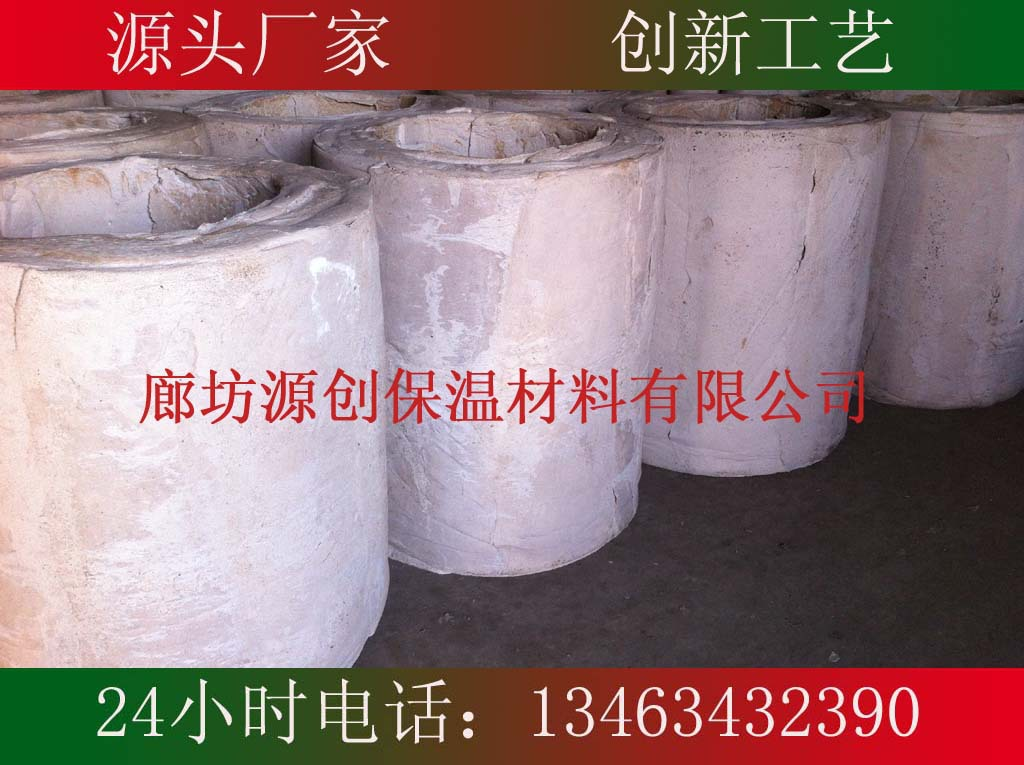r

r r r r r r r r r r r r r r r r r r r r r r r r r r r r r r r r r r r r r r r r r r r r r r r r r
 r r 项目r r r r 硅酸铝纤维管r r r r 异形制品r r r r 使用温度（℃）r r r r 1000r r r r 1260r r r r 体积密度（kg/m3）r r r r 100-180r r r r 150-300r r r r 导热r r r r 0.034（20℃）r r r r 0.127（600℃）r r r r 系数r r r r 0.09（400℃）r r r r 0.20（1000℃）r r r r (w/m.k)r r r r 0.12(600℃)r r r r  r r r r 渣球含量（%）>0.25mmr r r r 11r r r r 13.6r r r r 永久线收缩率%r r r r -3.5r r r r -2r r r r 保温24小时r r r r 600℃r r r r 1000℃r r
r

r  r

r

r  r

r

r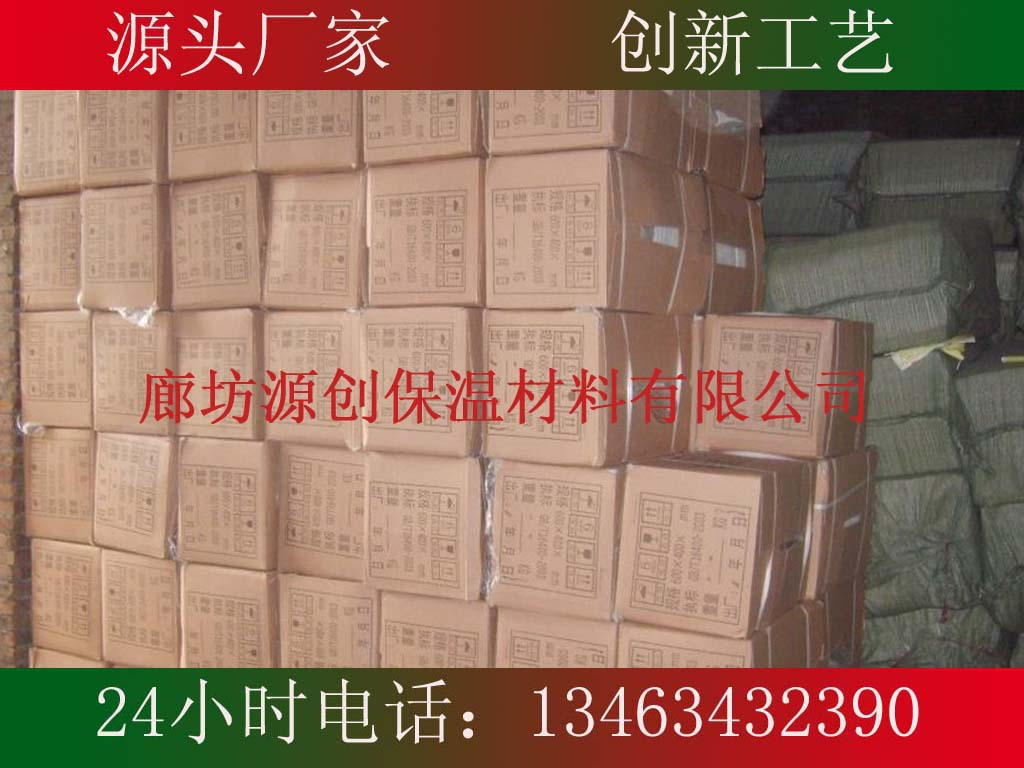r
r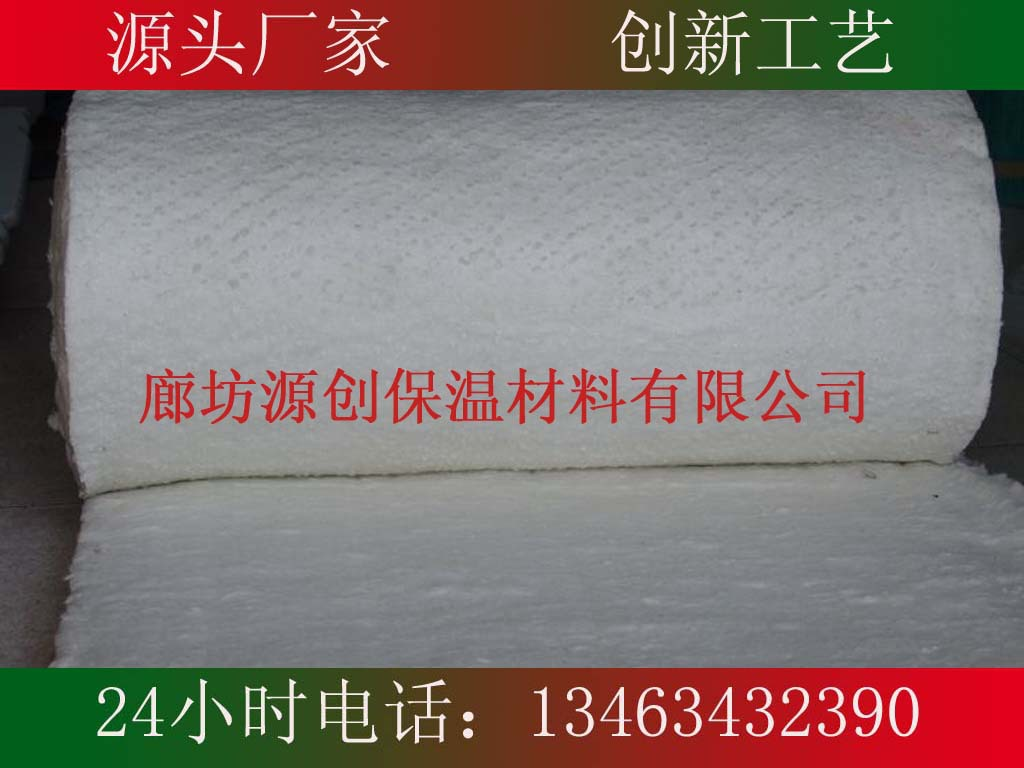r
r
r

r

r 硅酸铝毡
r 生产工艺
r   硅酸铝纤维毡，是以焦宝石为主要原料，在熔融、出丝（在出丝过程中加入适量粘合剂）、固化室固化、切割等工序制成。全过程由PLC自动控制，产品一次成型。
r

r

r  r

r

r  产品特性 r

r

r 1、低导热率、低热容量。
r 2、具有良好的绝热、吸音特性。
r 3、优良的化学稳定性及热稳定性。
r

r

r  r

r

r  主要规格
r 密度：80-130kg/m3  厚度：15-50mm  宽度：400、600、1200mm
r 长度：可根据用户要求定做。
r 根据不同用途分为：硅酸铝纤维卷毡、硅酸铝纤维平毡。
r

r

r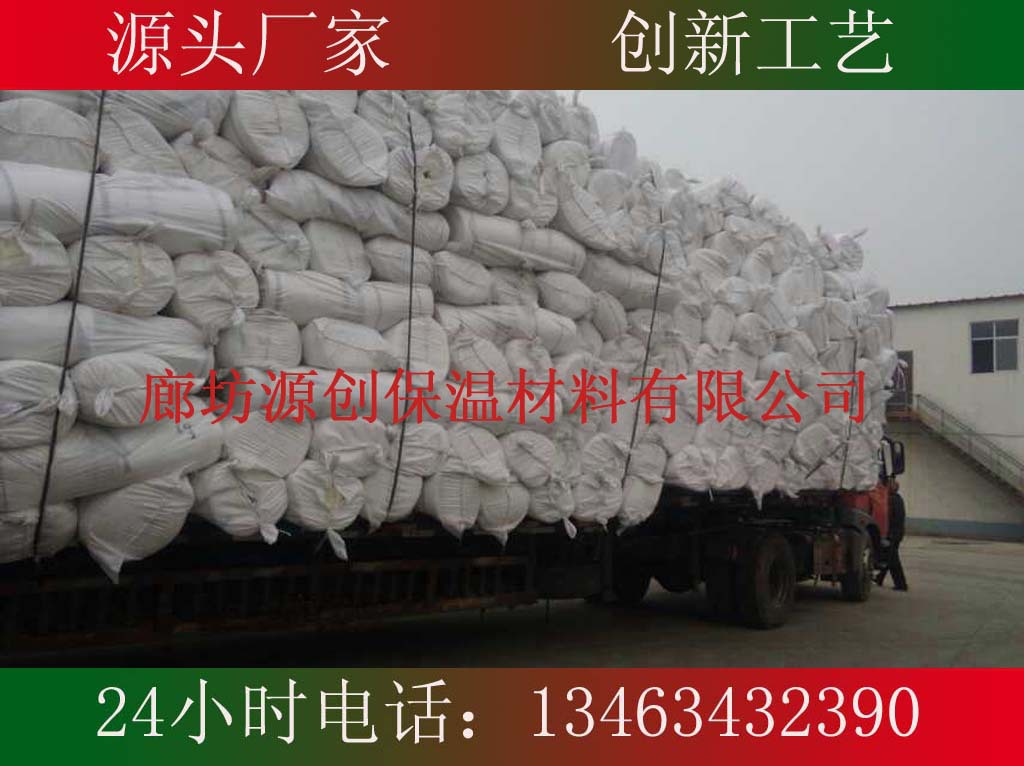r
r
r

r

r 硅酸铝针刺毯
r 品种类别
r    ※标准型硅酸铝针刺毯   ※高纯型硅酸铝针刺毯
r    ※高铝型硅酸铝针刺毯   ※含锆型硅酸铝针刺毯
r 生产工艺
r    采用先进的CWCN设备,双面针刺毯流水线,其设备与生产工艺均处在领先水平。各种硅酸铝纤维针刺毯，采用不同材质的原料（如焦宝石、SiO2、Al2O3、锆英砂等混配)所成纤维经沉降针刺、热定型、纵横切、打卷等工序制成。质地均匀，表面平整，具有优良的抗拉强度，因硅酸铝针刺毯本身不含粘结剂，所以大大提高了耐热程度。

r
r

r

r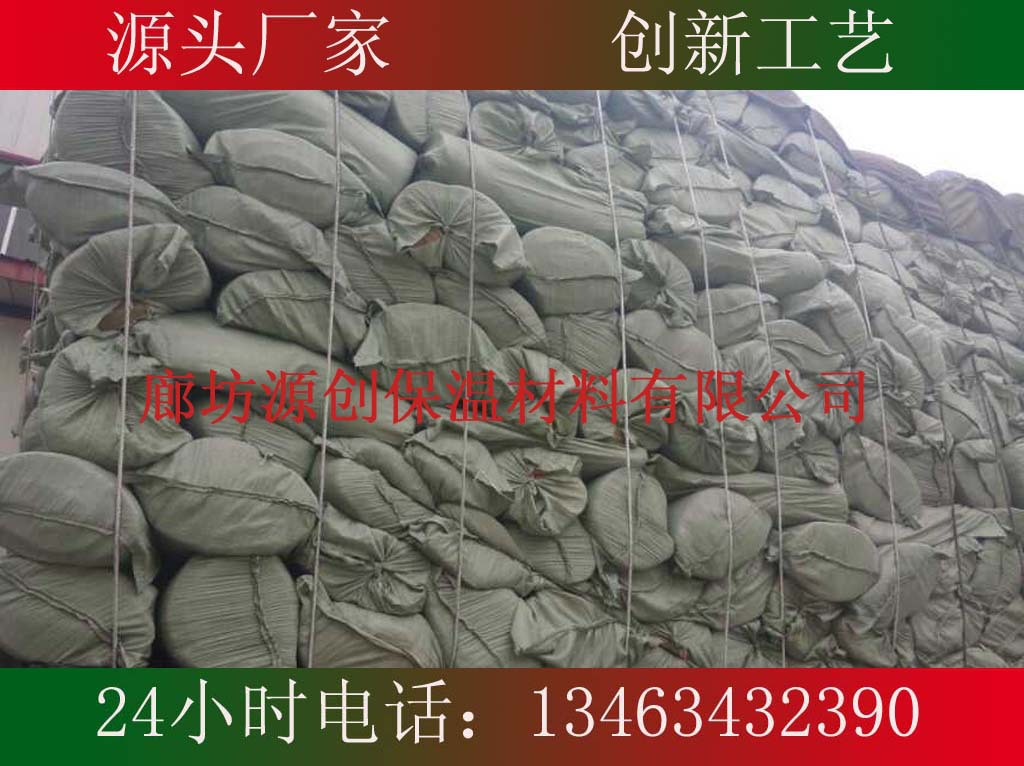r
r
r

r

r  r

r

r 如在购买中出现任何问题请及时联系我们的客服，或拨打我们的销售人员电话，我们会在第一时间为您解决。r

r

r  r

r

r 厂家销售 省去中间商环节，低价让利给客户r

r

r  r

r

r 材料规格：岩棉保温板 （规格型号可以定制）r

r

r 常规型号：厚30-150mm 长宽1200×600mm或1000×600mmr

r

r 密度：50-180kg/m3r

r

r  r

r r 售后说明r r
r
• r

r 物流配送r

r

r 1：可按客户要求提供最优适的配送方式。（零担、专线、配货）r

r

r 2：运费价格：按配送产品材质、数量及目的地具体核算，并承报客户。r

r
• r
• r
• r
• r

r 付款方式r

r
• r
• r

r 我公司支持在线支付，及现金转帐，电汇，方便客户下单。具体方式请在下单前与客户主管联系并确定。 r

r
• r
• r
• r
• r

r 退换货政策r

r
• r
• r

r 如果您在收货、施工使用的过程中遇到问题，请及时联系我们，我们的客服会给您专业的指导。如果收到货物后发现质量问题（非人为）需要 退货，经确认我们无条件接受退换货，并与亲协商。最后祝您购物愉快。 r

r
• r
r

r  r

r

r  r

0相关评论

## 相关栏目

### 按排行字母分类：

A B C D E F G H I J K L M N O P Q R S T U V W X Y Z